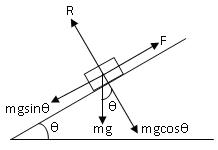Click to Chat

1800-1023-196

+91-120-4616500

CART 0

• 0

MY CART (5)

Use Coupon: CART20 and get 20% off on all online Study Material

ITEM
DETAILS
MRP
DISCOUNT
FINAL PRICE
Total Price: Rs.

There are no items in this cart.
Continue Shopping
        relationship between angle of friction and angle of repose
one year ago

							 I.e. angle of friction is equal to angle of repose.= i.e coefficient of limiting friction between any two surfaces in contact is equal to tangent of the angle of friction between them.Thus,  = tan= coefficient of limiting friction.This is equal to the tangent of the angle of repose between two surfaces in contact.Now, since  Where  = tan/mgcos ---------eq.2On dividing eq.1 by eq.2 we get,F/R = mgsin -------- eq.1R = mgcos opposite to FIn equilibrium,F = mgsin opposite to Rand mgsin Let us suppose a body is placed on an inclined plane as in the above figure.Various forces involved are:-1. weight,mg of the body,acting vertically downwards.2.normal reaction,R,acting perpendicular to inclined plane.3.Force of friction,F, acting up the plane.Now, mg can be resolved in two components:- mgcos and angle of repose = Suppose angle of friction =one year ago
							 I.e. angle of friction is equal to angle of repose.= i.e coefficient of limiting friction between any two surfaces in contact is equal to tangent of the angle of friction between them. Thus,  = tan= coefficient of limiting friction.This is equal to the tangent of the angle of repose between two surfaces in contact. Now, since  Where  = tan/mgcos ---------eq.2 On dividing eq.1 by eq.2 we get, F/R = mgsin -------- eq.1 R = mgcos opposite to F In equilibrium, F = mgsin opposite to R and mgsin Let us suppose a body is placed on an inclined plane as in the above figure. Various forces involved are:- 1. weight,mg of the body,acting vertically downwards.2.normal reaction,R,acting perpendicular to inclined plane. 3.Force of friction,F, acting up the plane. Now, mg can be resolved in two components:- mgcos and angle of repose = Suppose angle of friction =one year ago
							Sorry about the previous answers.... Solution: ,since coefficient of limiting friction between any two surfaces in contact is equal to tangent of the angle of friction between them.Thus, =coefficient of limiting friction)We know, (Therefore,   ---------eq.2On dividing eq.1 by eq.2 we get, -------- eq.1 opposite to FIn equilibrium, opposite to Rand Let us consider a body is placed on an inclined plane as in the above figure.Various forces involved are:-1. weight mg of the body, acting vertically downwards.2.normal reaction R, acting perpendicular to inclined plane.3.Force of friction F, acting up the plane.Now, mg can be resolved in two components:- and angle of repose = Let angle of friction =one year ago
Think You Can Provide A Better Answer ?

## Other Related Questions on Modern Physics

View all Questions »### Course Features

• 101 Video Lectures
• Revision Notes
• Previous Year Papers
• Mind Map
• Study Planner
• NCERT Solutions
• Discussion Forum
• Test paper with Video Solution### Course Features

• 110 Video Lectures
• Revision Notes
• Test paper with Video Solution
• Mind Map
• Study Planner
• NCERT Solutions
• Discussion Forum
• Previous Year Exam Questions